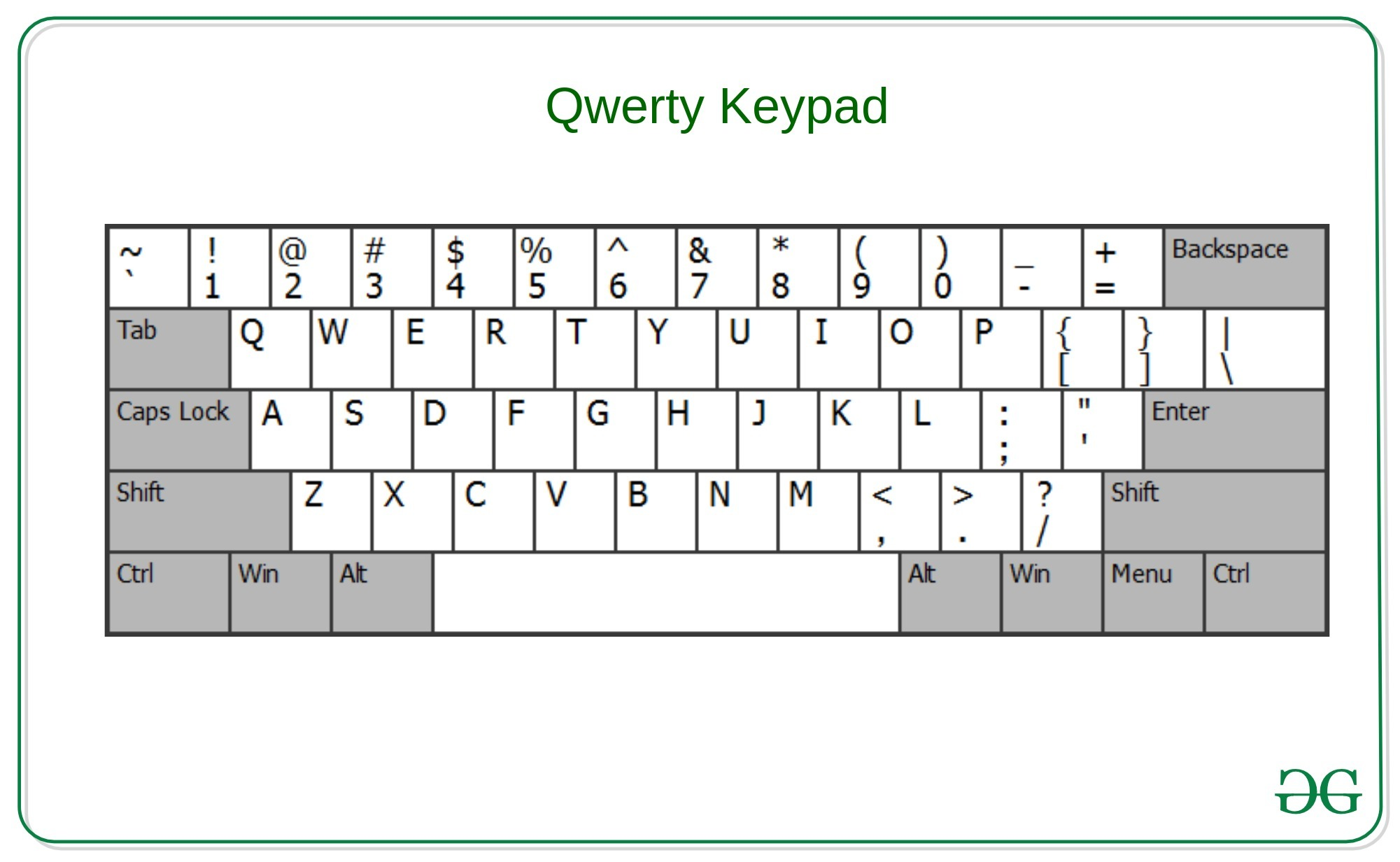# Check whether the string can be printed using same row of qwerty keypad

Given a string S, the task is to check whether the string can be typed using only a single row of the qwerty keyboard.Examples:

Output: Yes
Explanation:
Characters “D” and “a” are present in the same row of qwerty keypad. That is second Row.

Input: S = “Mom”
Output: No
Explanation:
Characters “M” and “o” are not present in the same row of qwerty keypad.

## Recommended: Please try your approach on {IDE} first, before moving on to the solution.

Approach: The idea is to store the characters of the same row of the qwerty keypad into different hash-maps to check that all the characters of the string are from the same row.

Below is the implementation of the above approach:

## C++

 `// C++ Program to check whether ` `// the string can be printed ` `// using same row of qwerty keypad ` ` `  `#include ` `using` `namespace` `std; ` ` `  `// Function to find the row of the ` `// character in the qwerty keypad ` `int` `checkQwertyRow(``char` `x) ` `{ ` `    ``// Sets to include the characters ` `    ``// from the same row of the qwerty keypad ` `    ``set<``char``> first_row ` `        ``= { ``'1'``, ``'2'``, ``'3'``, ``'4'``, ` `            ``'5'``, ``'6'``, ``'7'``, ``'8'``, ` `            ``'9'``, ``'0'``, ``'-'``, ``'='` `}; ` `    ``set<``char``> second_row ` `        ``= { ``'Q'``, ``'W'``, ``'E'``, ``'R'``, ``'T'``, ` `            ``'Y'``, ``'U'``, ``'I'``, ``'O'``, ``'P'``, ` `            ``'['``, ``']'``, ``'q'``, ``'w'``, ``'e'``, ` `            ``'r'``, ``'t'``, ``'y'``, ``'u'``, ``'i'``, ` `            ``'o'``, ``'p'` `}; ` `    ``set<``char``> third_row ` `        ``= { ``'A'``, ``'S'``, ``'D'``, ``'F'``, ``'G'``, ` `            ``'H'``, ``'J'``, ``'K'``, ``'L'``, ``';'``, ` `            ``':'``, ``'a'``, ``'s'``, ``'d'``, ``'f'``, ` `            ``'g'``, ``'h'``, ``'j'``, ``'k'``, ``'l'` `}; ` `    ``set<``char``> fourth_row ` `        ``= { ``'Z'``, ``'X'``, ``'C'``, ``'V'``, ``'B'``, ` `            ``'N'``, ``'M'``, ``','``, ``'.'``, ` `            ``'/'``, ``'z'``, ``'x'``, ``'c'``, ``'v'``, ` `            ``'b'``, ``'n'``, ``'m'` `}; ` ` `  `    ``// Condition to check the row of the ` `    ``// current character of the string ` `    ``if` `(first_row.count(x) > 0) { ` `        ``return` `1; ` `    ``} ` `    ``else` `if` `(second_row.count(x) > 0) { ` `        ``return` `2; ` `    ``} ` `    ``else` `if` `(third_row.count(x) > 0) { ` `        ``return` `3; ` `    ``} ` `    ``else` `if` `(fourth_row.count(x) > 0) { ` `        ``return` `4; ` `    ``} ` ` `  `    ``return` `0; ` `} ` ` `  `// Function to check the characters are ` `// from the same row of the qwerty keypad ` `bool` `checkValidity(string str) ` `{ ` `    ``char` `x = str; ` `    ``int` `row = checkQwertyRow(x); ` `    ``for` `(``int` `i = 0; i < str.length(); i++) { ` `        ``x = str[i]; ` `        ``if` `(row != checkQwertyRow(x)) { ` `            ``return` `false``; ` `        ``} ` `    ``} ` `    ``return` `true``; ` `} ` ` `  `// Driver Code ` `int` `main() ` `{ ` `    ``string str = ``"GeeksforGeeks"``; ` ` `  `    ``if` `(checkValidity(str)) ` `        ``cout << ``"Yes"``; ` `    ``else` `        ``cout << ``"No"``; ` ` `  `    ``return` `(0); ` `} `

## Java

 `// Java program to check whether ` `// the string can be printed ` `// using same row of qwerty keypad ` `import` `java.util.*; ` ` `  `class` `GFG{ ` ` `  `// Function to find the row of the ` `// character in the qwerty keypad ` `static` `int` `checkQwertyRow(``char` `x) ` `{ ` `     `  `    ``// Sets to include the characters ` `    ``// from the same row of the qwerty keypad ` `    ``Character[] first_row1 = { ``'1'``, ``'2'``, ``'3'``, ``'4'``, ` `                               ``'5'``, ``'6'``, ``'7'``, ``'8'``, ` `                               ``'9'``, ``'0'``, ``'-'``, ``'='` `}; ` `    ``Set first_row = ``new` `HashSet<>( ` `     ``Arrays.asList(first_row1)); ` `      `  `    ``Character[] second_row1 = { ``'Q'``, ``'W'``, ``'E'``, ``'R'``, ``'T'``, ` `                                ``'Y'``, ``'U'``, ``'I'``, ``'O'``, ``'P'``, ` `                                ``'['``, ``']'``, ``'q'``, ``'w'``, ``'e'``, ` `                                ``'r'``, ``'t'``, ``'y'``, ``'u'``, ``'i'``, ` `                                ``'o'``, ``'p'` `}; ` `    ``Set second_row = ``new` `HashSet<>( ` `     ``Arrays.asList(second_row1)); ` `      `  `    ``Character[] third_row1 = { ``'A'``, ``'S'``, ``'D'``, ``'F'``, ``'G'``, ` `                               ``'H'``, ``'J'``, ``'K'``, ``'L'``, ``';'``, ` `                               ``':'``, ``'a'``, ``'s'``, ``'d'``, ``'f'``, ` `                               ``'g'``, ``'h'``, ``'j'``, ``'k'``, ``'l'` `}; ` `    ``Set third_row = ``new` `HashSet<>( ` `     ``Arrays.asList(third_row1)); ` `      `  `    ``Character[] fourth_row1 = { ``'Z'``, ``'X'``, ``'C'``, ``'V'``, ``'B'``, ` `                                ``'N'``, ``'M'``, ``','``, ``'.'``, ` `                                ``'/'``, ``'z'``, ``'x'``, ``'c'``, ``'v'``, ` `                                ``'b'``, ``'n'``, ``'m'` `}; ` `    ``Set fourth_row = ``new` `HashSet<>( ` `     ``Arrays.asList(fourth_row1)); ` ` `  `    ``// Condition to check the row of the ` `    ``// current character of the string ` `    ``if` `(first_row.contains(x))  ` `    ``{ ` `        ``return` `1``; ` `    ``} ` `    ``else` `if` `(second_row.contains(x)) ` `    ``{ ` `        ``return` `2``; ` `    ``} ` `    ``else` `if` `(third_row.contains(x))  ` `    ``{ ` `        ``return` `3``; ` `    ``} ` `    ``else` `if` `(fourth_row.contains(x))  ` `    ``{ ` `        ``return` `4``; ` `    ``} ` `    ``return` `0``; ` `} ` ` `  `// Function to check the characters are ` `// from the same row of the qwerty keypad ` `static` `boolean` `checkValidity(String str) ` `{ ` `    ``char` `x = str.charAt(``0``); ` `    ``int` `row = checkQwertyRow(x); ` `     `  `    ``for``(``int` `i = ``0``; i < str.length(); i++)  ` `    ``{ ` `        ``x = str.charAt(i); ` `        ``if` `(row != checkQwertyRow(x)) ` `        ``{ ` `            ``return` `false``; ` `        ``} ` `    ``} ` `    ``return` `true``; ` `} ` ` `  `// Driver code ` `public` `static` `void` `main(String[] args) ` `{ ` `    ``String str = ``"GeeksforGeeks"``; ` ` `  `    ``if` `(checkValidity(str)) ` `        ``System.out.println(``"Yes"``); ` `    ``else` `        ``System.out.println(``"No"``); ` `} ` `} ` ` `  `// This code is contributed by offbeat `

Output:

```No
```

Time Complexity: O(N)

Attention reader! Don’t stop learning now. Get hold of all the important DSA concepts with the DSA Self Paced Course at a student-friendly price and become industry ready.

My Personal Notes arrow_drop_upCheck out this Author's contributed articles.

If you like GeeksforGeeks and would like to contribute, you can also write an article using contribute.geeksforgeeks.org or mail your article to contribute@geeksforgeeks.org. See your article appearing on the GeeksforGeeks main page and help other Geeks.

Please Improve this article if you find anything incorrect by clicking on the "Improve Article" button below.

Improved By : offbeat